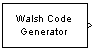# Walsh Code Generator

Generate Walsh code from orthogonal set of codes

## Library

Sequence Generators sublibrary of Comm Sources

•## Description

Walsh codes are defined as a set of N codes, denoted Wj, for j = 0, 1, ... , N - 1, which have the following properties:

• Wj takes on the values +1 and -1.

• Wj = 1 for all j.

• Wj has exactly j zero crossings, for j = 0, 1, ... , N - 1.

• ${W}_{j}{W}_{k}^{T}=\left\{\begin{array}{cc}0& j\ne k\\ N& j=k\end{array}$

• Each code Wj is either even or odd with respect to its midpoint.

Walsh codes are defined using a Hadamard matrix of order N. The Walsh Code Generator block outputs a row of the Hadamard matrix specified by the Walsh code index, which must be an integer in the range [0, ..., N - 1]. If you set Walsh code index equal to an integer j, the output code has exactly j zero crossings, for j = 0, 1, ... , N - 1.

Note, however, that the indexing in the Walsh Code Generator block is different than the indexing in the Hadamard Code Generator block. If you set the Walsh code index in the Walsh Code Generator block and the Code index parameter in the Hadamard Code Generator block, the two blocks output different codes.

## Parameters

Code length

Integer scalar that is a power of 2 specifying the length of the output code.

Code index

Integer scalar in the range [0, 1, ... , N - 1], where N is the Code length, specifying the number of zero crossings in the output code.

Sample time

Positive scalars specify the time in seconds between each sample of the output signal. If you set the Sample time to `-1`, the output signal inherits the sample time from downstream. For information on the relationship between the Sample time and Samples per frame parameters, see Sample Timing.

Samples per frame

Samples per frame, specified as a positive integer indicating the number of samples per frame in one channel of the output data. If Samples per frame is greater than the Code length, the code is cyclically repeated. For information on the relationship between Sample time and Samples per frame, see Sample Timing.

Output data type

The output type of the block can be specified as an `int8` or `double`. By default, the block sets this to `double`.

expand all

## Compatibility Considerations

expand all

Behavior changed in R2020a